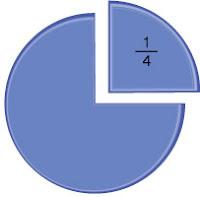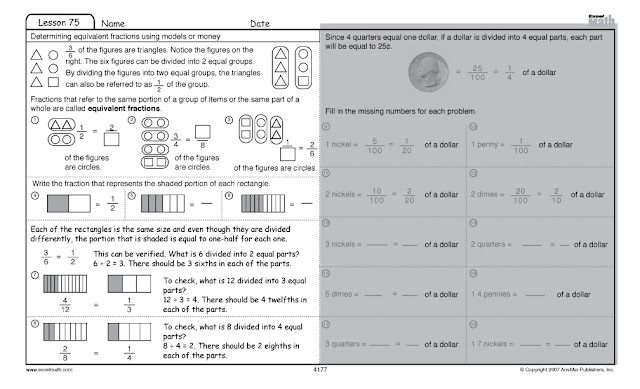## Wednesday, April 11, 2012

### Recognizing Equivalent Representations of Fractions

In mathematics, we can represent numbers in a variety of ways. So one-fourth can be notated as a decimal: .25

or as a fraction:

or as a colored box:

or as various shapes:

or as a pie chart:or even as an actual pie with pi:

In Excel Math, students learn "to recognize equivalent representations for the same number and generate them by decomposing and composing numbers ..."

Fractions that refer to the same portion of a group of items or the same part of a whole are called equivalent fractions.

Let's take a look at an Excel Math Fourth Grade Student Lesson Sheet to find out how to determine equivalent fractions using models. (Next week we'll look at money.) Here's a good explanation of how to determine equivalent fractions:Excel Math Fourth Grade Lesson 75 Student Worksheet
In problem number 1 there are four figures shown (two triangles and two circles). Two of the four figures are circles.
Here's another way to say it:

In this fraction, the numerator (number above the fraction line) is 2  and the denominator (number below the line) is 4. So 4 goes in the empty box to complete the equation:

You can help your students remember the difference between numerator and denominator by pointing out that the denominator is down below the fraction line and both denominator and down begin with "d." For a complete Glossary of Math Terms for Kindergarten through Sixth Grade (in English or Spanish), visit our website.

Feel free to print this student worksheet and try it out with your students. Click here for a PDF file you can download. (However, since Excel Math uses a unique spiraling strategy, you will need to teach multiple lessons sequentially within each grade level in order to get the concepts into your students' long-term memory.)Excel Math Fourth Grade Lesson 75 Student Worksheet Click here for a free math worksheet you can print out and use today.

Next week we'll take a look at the monetary equivalents shown on the right-hand side of this worksheet.